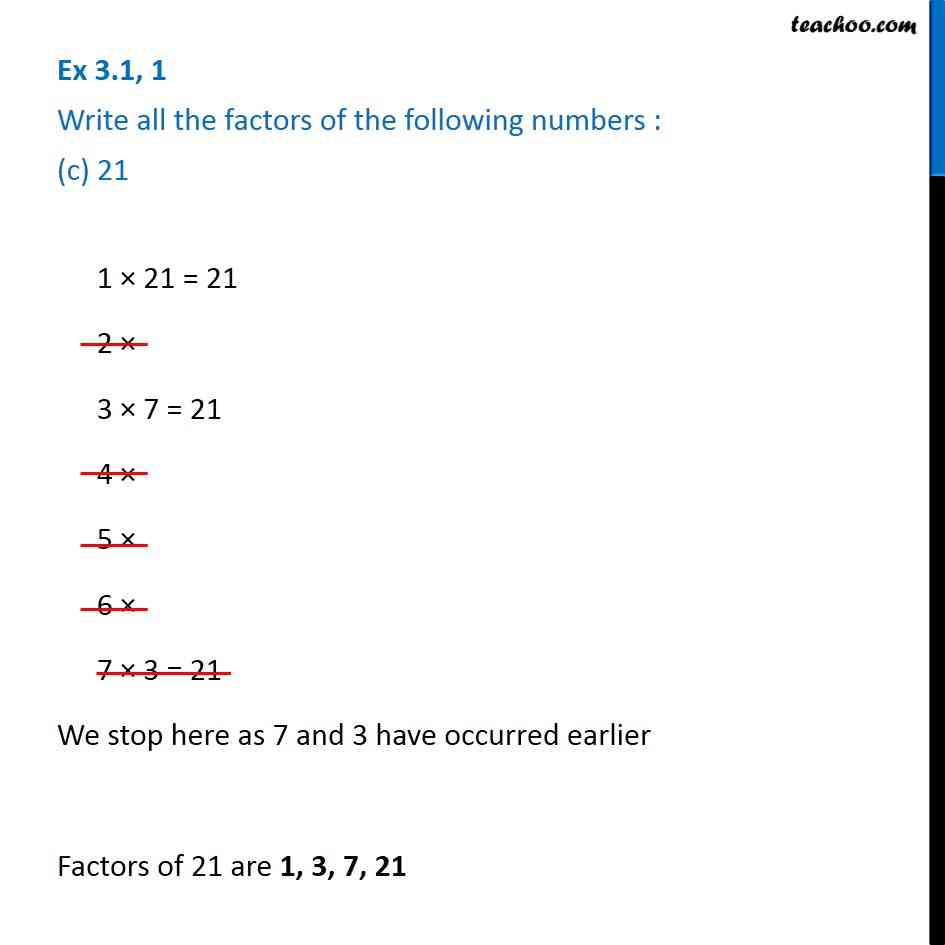1. Chapter 3 Class 6 Playing with Numbers
2. Serial order wise
3. Ex 3.1

Transcript

Ex 3.1, 1 Write all the factors of the following numbers : (c) 21 1 × 21 = 21 2 × 3 × 7 = 21 4 × 5 × 6 × 7 × 3 = 21 We stop here as 7 and 3 have occurred earlier Factors of 21 are 1, 3, 7, 21